# RF Tutorial Lesson 7: Designing Distributed Bandpass Filters Using Coupled Transmission Line Segments

 Tutorial Project: Designing Distributed Bandpass Filters Using Coupled Transmission Line Segments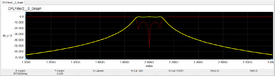Objective: In this project, you will build a distributed bandpass filter using distributed coupled transmission line segments. Concepts/Features: Coupled Transmission Lines Even Mode Odd Mode System Characteristic Impedance Network Analysis Minimum Version Required: All versions

## What You Will Learn

In this tutorial first you will cascade several sections of generic coupled T-Lines to design a distributed bandpas filter. Then, you will realize a microstrip version of your distributed filter.

## Examining the Filter Building Block

A two-port network can be formed by terminating two of the four ports of a Generic Coupled T-Lines device in either short or open circuits. Several configurations are possible. You can then build narrowband bandpass filters by cascading several of such terminated coupled transmission line segments. For this project, you will open-circuit Ports 2 and 3 of the Generic Coupled T-Lines device and will use Port 1 and Port 4 as the input and output ports of the resulting two-port network, respectively. This is shown in the figure below: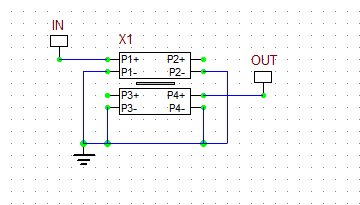The coupled line building block for the distributed bandpass filter.

In this case, the 4×4 Z matrix is reduced to a 2×2 matrix with the following elements:

$Z_{11} = Z_{22} = -\frac{j}{2} \left( Z_{0e} + Z_{0o} \right) cot \left( \frac{2\pi f}{c} L \sqrt{\epsilon_{eff}} \right)$

$Z_{21} = Z_{12} = -\frac{j}{2} \left( Z_{0e} - Z_{0o} \right) csc \left( \frac{2\pi f}{c} L \sqrt{\epsilon_{eff}} \right)$

The following is a list of parts needed for this part of the tutorial lesson:

Part Name Part Type Part Value
VS1 - VS2 AC Voltage Source 1V
X1 Generic Coupled T-Lines Z0e = 70, Z0o = 30, len = 37.5
IN Net Marker N/A
OUT Net Marker N/A

Build a new circuit using the Generic Coupled T-Lines device and use two Net Markers to designate the input and output ports. Leave Ports 2 and 3 of the device X1 open as shown in the above figure.

Run a Network Analysis Test of your circuit with the parameters specified in the table below. Make sure to uncheck the "Decibels" checkbox.

 Start Frequency 1G 3G 1G Linear Z Cartesian (Real/Imag)

The simulated Z-parameter results are shown below and then compared to their analytical values in the second table: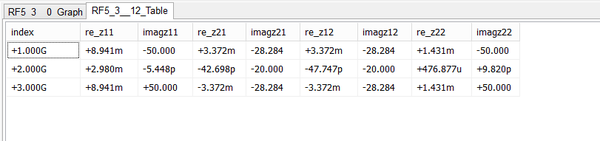Real and imaginary parts of the Z11 and Z21 parameters of the open-circuited Generic Coupled T-Line two-port.
f βL Analytical Z11 [Ω] Simulated Z11 [Ω] Analytical Z21 [Ω] Simulated Z21 [Ω]
1GHz π/4 -j50 0.009 - j50 -j28.284 0.003 - j28.284
2GHz π/2 0.003 0 -j20 - j20
3GHz 3π/4 j50 0.009 + j50 -j28.284 -0.003 - j28.284

## Designing a Coupled Line Bandpass Filter

In this part of the tutorial lesson you will cascade four quarter-wavelength Generic Coupled T-Line segments to build a distributed bandpass filter as shown in the opposite figure. Port 1 of the first segment and Port 4 of the last segment are designated as the input and output ports. Port 4 of each segment is fed into Port 1 of the next segment. Ports 2 and 3 of all coupled line devices are left open-circuited. Proper grounding is done for all the negative pins.The schematic of the generic coupled line bandpass filter.

The following table gives the parameters of each coupled line segment:

Coupled Line Segment Z0e Z0o eeff len
L1 70.61Ω 39.24Ω 1 37.5mm
L2 56.64Ω 44.77Ω 1 37.5mm
L3 56.64Ω 44.77Ω 1 37.5mm
L4 70.61Ω 39.24Ω 1 37.5mm

All the segment lengths are chose to be len = 37.5mm, which is a quarter wavelength at the center frequency of the filter fo = 2GHz. TEM transmission lines with εeff = 1 are assumed. The impedances have been chosen to achieve an equal-ripple bandpass filter design.

Run a network analysis of this two-port circuit with the parameters specified below:

 Start Frequency 1G 3G 10Meg Linear S Cartesian (Amplitude Only) with Decibels

The figure below shows the graph of S11 and S21 parameters. Note that a linear scale is used for frequency (the bottom axis). The filter has a center frequency of fo = 2GHz with a 3dB bandwidth of about 235MHz. The response drops to -45dB at 1.5GHz and 2.5GHz.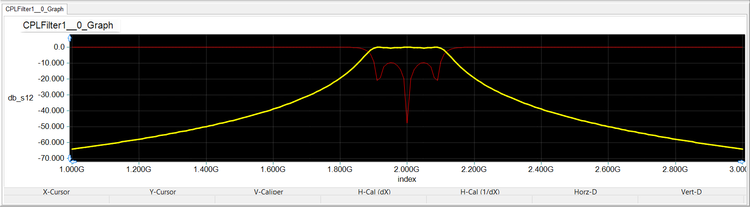Graph of S11 and S21 parameters of the distributed Generic Coupled T-Line filter over the frequency range 1GHz-3GHz.

## Designing Two Sets of Coupled Microstrip Lines

In this part of the tutorial lesson, you will design and test a microstrip realization of the coupled line bandpass filter you simulated in the previous part. For this purpose, you will use a substrate of thickness h = 1.6mm with εeff = 3.4. You will assume a lossless substrate (tand = 0). Remember that in the previous section, TEM line segments with εeff = 1 were assumed and the length of the coupled line segments were set to be a quarter free-space wavelength at 2GHz. For this part, first you need to design coupled microstrip lines with the given even and odd mode impedances. Then, you have to calculate the guide wavelengths of the couple microstrips at 2GHz. You will do these using RF.Spice's Device Manager.

Open the Coupled Microstrips Designer dialog from the Tools Menu of Device Manager. Enter the substrate parameters: h = 1.6mm and er = 3.4. Enter the Z0e and Z0o values for the two types of coupled line segments from the previous section and calculate width and spacing of the coupled microstrip lines for each case. Then, open the Coupled Microstrips Calculator dialog from the Tools Menu of Device Manager. Enter the calculated microstrip width and spacing values to verify your design and also find the corresponding guide wavelengths λg at 2GHz. The lengths of the coupled microstrip segments are chosen to be a quarter of the corresponding guide wavelength at 2GHz. The following table summarizes the parameters of the coupled microstrip segments L1, L2, L3 and L4.

Coupled Microstrip Segment Z0e Z0o w s Average εeff λg at 2GHz len
L1 70.61Ω 39.24Ω 2.96mm 0.4mm 2.57 93.52mm 23.38mm
L2 56.64Ω 44.77Ω 3.6mm 1.68mm 2.66 91.97mm 23mm
L3 56.64Ω 44.77Ω 3.6mm 1.68mm 2.66 91.97mm 23mm
L4 70.61Ω 39.24Ω 2.96mm 0.4mm 2.57 93.52mm 23.38mmRF.Spice's Coupled Microstrips Designer dialog.RF.Spice's Coupled Microstrips Calculator dialog.

## Realizing the Microstrip Version of the Coupled Line Bandpass Filter

Build your microstrip circuit using four "Coupled Mirostrips" parts with the parameters specified in the above table. You can access the part from Menu > Transmission Lines > Physical Transmission Lines > Coupled Microstrips. Connect the four parts in a cascaded fashion as shown in the figure below.The schematic of the coupled microstrip bandpass filter.

Run a network analysis of this two-port circuit with the same parameters as in the previous section:

 Start Frequency 1G 3G 10Meg Linear S Cartesian (Amplitude Only) with Decibels

The graphs of S11 and S21 parameters are shown in the figure below and agree perfectly with the results of the previous section with the generic coupled T-Lines.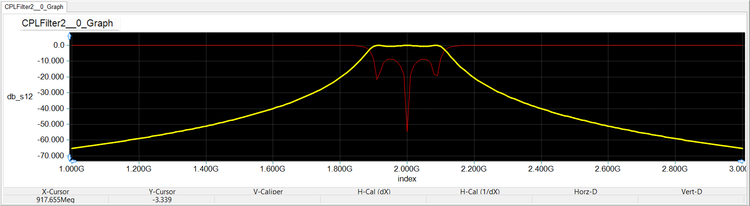Graph of s11 and s21 parameters of the Coupled Microstrip bandpass filter over the frequency range 1GHz-3GHz.